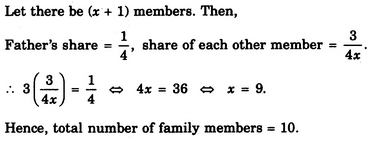# Verbal Reasoning - Arithmetic Reasoning - Discussion

### Discussion :: Arithmetic Reasoning - Section 1 (Q.No.43)

43.

In a family, the father took 1/4 of the cake and he had 3 times as much as each of the other members had. The total number of family members is

 [A]. 3 [B]. 7 [C]. 10 [D]. 12

Explanation:Suresh said: (Apr 1, 2013) How there could be (x+1) members why can't x members?

 Apeksha said: (Aug 24, 2013) Suppose there is 100 kg of cake, then father's share = 25kg. So each family member will get 25/3 kg. Let x be the no.of members. Therefore, x(25/3) = 75. x = 9. Total members 9+1(father).

 Teja said: (Oct 20, 2014) Let total number of family members be a. Total amount of cake be x. 1/4x is fathers part. 3/4x is remaining members part (a-1). 1/4x = 3(3/4x)/a-1 (remaining members excluding father). a-1 = 9. a = 10.

 Ravi. U S said: (May 3, 2017) This type of problem can be solved still easier way. Father had 1 part of a cake in 4 parts. Remaining parts are 3. Multiply the remaining parts with the number of times it is more. As given in the problem 3*3= 9. Including father, it is 10 members.

 Gauri said: (Sep 21, 2017) Nice @Ravi.

 Arjun said: (Apr 2, 2018) Let the family members b x. Father ate 1/4 of the cake; Rest members got 3/4x, ATQ, the father had 3 times more than other members share i.e 3/4, Now, 3(3/4x) = 1/4, 3/4x = 1/12, 36 = 4x, X = 9, 9 + 1(father) = 10 total family member.

 Tharini said: (Jun 30, 2019) Good explanation. Thanks @Ravi.

 Kanchik said: (Dec 11, 2019) @Ravi suberb.

 Kavi said: (Oct 23, 2020) Thanks @Ravi.

 Rakesh said: (May 20, 2021) Nice, thanks @Ravi.

 Dhruvan said: (Jul 14, 2021) @Arjun. Why take x for 3/4 term and not for 1/4 term? I think x term will be both sides of the eqn.

 Isa said: (Oct 14, 2021) As per the question, each and every other 1/4 of the cake was shared by 3 people as compared to that of their father. Remaining quarters are 3*3(people) for all 3 quarters = 9 + 1(Father) = 10.